# An Introduction to Binary Trees

## Introduction

A Binary Trees on a computing level is used to store data in a hierarchical manner, which will help in sorting and searching data efficiently. Before introducing binary trees, let us first look into what M-ary trees are.

## M-ary Trees

M-ary tree is a rooted tree where every node has at most m children (i.e. <= m).  A tree is a full m-ary tree if every internal node has m children.

## Introduction to Binary trees data structure

A Binary tree is an M-ary tree with m = 2.

Binary trees are a particular type of tree where each internal node have at most two children.

A Binary Tree can also be defined as a tree where each vertex has a degree of at most 2.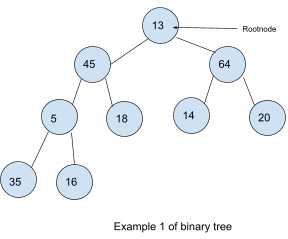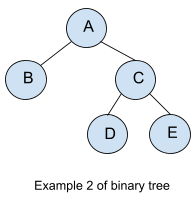The root node divides the tree into two subtrees:-

• Left sub-tree
• Right sub-tree

In Example 1, the data 45, 5, 18, 35 and 16 form the left subtree and 64, 14 and 20 form the right sub-tree of root node 13.

In Example 2, data B form the left subtree and data C, D and E form the right subtree of root node A.

Any node N in a binary tree can have 0, 1 or 2 successors.

## Properties of a binary tree.

• If ‘ l ’ is the level of a binary tree, the maximum number of nodes on any level in a binary tree is 2l.

• For example, the root node is at level 0, so the number of nodes at the root is 20 = 1.
• The children of the root node are at level 1, so the maximum number of nodes at level 1 is 21 = 2, and so on.
• This can be proved by mathematical induction.

• The maximum number of nodes possible in a tree of height h is 2h- 1

• Here the root node is considered to be at the height of 1. ( In some books, the height of the root node is considered to be at the height of 0. Here, the formula is considered as 2h+1- 1.)
• For example, the root node is at the height of 1. So 21- 1 = 1 node, which is true.
• The children of the root node are at the height of 2. So 22- 1 = 3 nodes, which is true.
• This can be proved by mathematical induction.

• The minimum number of nodes in a binary tree of height h  is ‘ h ‘.

• For example, the root node is at level 1, so the minimum number of nodes at level 1 is 1.
• Since the minimum number of nodes at the first level, there can be only one node whose height is 2. So the minimum number of nodes at the height of 2 is 2.

• For a non-empty binary tree, if “n” is the number of edges and “e” is the number of edges, then n = e+1

• In the above example, there are 15 nodes, so the number of edges e = 15+1=16 is valid.

## Types of binary tree

### Complete Binary Tree

A binary tree is a complete binary tree if, at all its levels except the last level, it has the maximum number of nodes, and nodes at the previous level appear as left as possible.

### Full Binary Tree

A binary tree is a full binary tree in which each node has either 0 or 2 children.

### Perfect Binary Tree

A binary tree is a perfect binary tree in which all the internal nodes have two children, and all the leaf nodes are at the same level.

### Degenerate Tree

Binary Trees where every parent node has one child, and it can be either left or right.

### Skewed trees

A binary tree where every parent node has only one child and that child should be strictly either left or right of every parent node.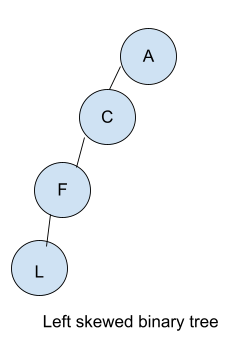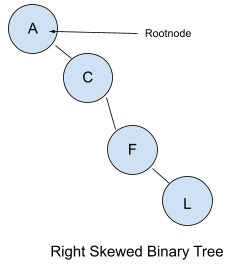## Representation of binary tree

### Array representation (Implicit Representation )

Array representation of a binary tree is static representation. Here size of the array is declared beforehand, which is a massive disadvantage because if we declare an array of size 10, then after 10, no more elements can be added to the tree, even if we want to. Anyway, let us see how elements of a tree are stored in an array.

Consider a tree a shown below:-

Here the numbers on top of each node represent their respective positions in the array. When these elements of the tree are arranged in an array, the height of it will look like:-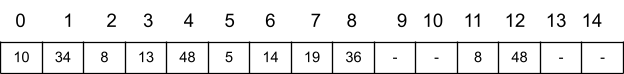For any node with index i, 1< i <= n

• Left Child ( i ) = 2*i + 1
• Right Child( i ) = 2*i + 2

Binary trees were created for efficient insertion, deletion and traversal operations, but implementing binary trees with an array is quite costly, so we have another representation of tree called the linked representation of binary tree ( Note:- This is not Linked list, as the Linked list is a linear data structure )

### Linked representation ( Explicit Representation )

This linked representation is the most efficient representation of the binary tree. For the linked representation of a binary tree, we use the concept of a doubly linked list.

In the above figure, a node with data 10 is represented in linked representation. Here LC represents the address of the left child, and RC represents the address of the right child, respectively.

So, the structure of a node in linked representation is as follows:-

So a binary tree representation using linked representation is as follows:-

In the linked representation of binary trees, we create a node whenever it is necessary. It is like the dynamic insertion of a node, whereas in an array, it is static, so a linked list representation of binary trees is advantageous.

## FAQs

1. What are Binary Trees?
Binary Trees are trees in which each node has at most two children.

2. What are the different types of Binary Trees?
Different types of binary trees are:-
Complete Binary Tree
Full Binary Tree
Skewed Binary Tree
Perfect Binary Tree
Degenerate Binary Trees

3. What is the advantage of using a binary tree?
The advantages are it helps in the storage of data in a hierarchical manner which helps in easy traversal operation, and it makes insertion and deletion faster than the arrays.

4. What are the time and space complexities of using binary trees?
The time and space complexities of a binary tree are O( n ).

5. Where are the uses of binary trees?
The primary use of binary trees is to search and sort as the data is stored hierarchically.

## Key Takeaways

So to summarise all you read, it covers topics about m-ary trees. We have looked at what a binary tree is, then the different properties of a binary tree, and how a binary tree is represented using arrays and linked lists. Further, we have seen why using an array for a binary tree is inefficient and how linked representation of a binary tree helps in overcoming the difficulties faced by the array.

Binary trees are an essential data structure that needs to be mastered to ace coding interviews. Check out the course Basics of C++ with Data Structures and Algorithms offered by Coding Ninjas to fully understand binary trees and other data structures.

Happy learning!.